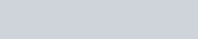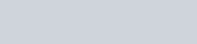ChemTalk## Consecutive Reaction Kinetics without the Steady State Approximation

The steady state approximation is a useful tool to simplify the math modeling a consecutive reaction mechanism. In chemical kinetics, consecutive reactions may seem to have straightforward kinetics but often involve some headache-inducing math. Take the following consecutive reaction: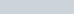Molecule A converts into B, which in turn converts into C. Rate constants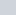and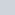inform the rates of the first and second reactions, respectively. Since each reaction is unimolecular, we can easily find the rates of change of each species in the reaction:Using the integrated first-order rate law, we can also find the equation modeling the concentration of A over time: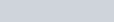We can then substitute this rate law into our rate of change in B: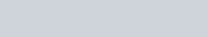We can solve this differential equation if we assume that there was no initial concentration of B (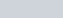and that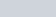: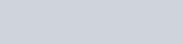Finally, if we also assume that there was no initial concentration of C (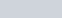,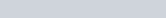), we then get an equation for the concentration of C over time: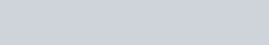As you can see, using fairly straightforward concepts of first-order kinetics, the math for consecutive reactions gets complicated fast. When you consider reactions with more than two steps, the math becomes unmanageable. This is where the steady state approximation proves useful.

## Steady State Approximation for Unimolecular Kinetics

The steady state approximation holds that the change in concentration of molecule B, the intermediate compound, always remains very low, if not negligible, at any point in time.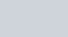To make this approximation , the consecutive reaction system must meet two often-true criteria:

• The concentration of B generally remains much lower relative to A or C.
• The rate of A reacting to form B is much slower than that of B reacting to form C. This means A forming B is the rate determining step.

Many consecutive reaction systems meet these criteria. Often, B behaves as an unstable intermediate, causing it to initially rise in concentration until meeting a certain low threshold. Then, any additional B produced rapidly converts to C much faster than the production of B due to its instability. Mathematically, This means thatrepresents a much higher quantity than.Graph of a system under the steady state approximation. Notice how the concentration of B stays low and changes little.

## Applying the Steady State Assumption

With both criteria met, the steady state approximation drastically simplifies the math. Namely, it gives us a way to relate the concentrations of A and B directly:This allows us to more easily arrive at an expression for the concentration of C as a function of time:Then, we implement the first-order rate law relating to change in A to finally express C in terms of time:Often, we can simplify the equation further, ifis negligible compared to(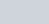). This allows us to neglect the third term in the parenthesis, yielding: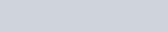## Steady State Approximation for Enzyme Kinetics

In addition to unimolecular kinetics, the steady state approximation proves useful in more complicated consecutive reactions. The most important application of the approximation in non-unimolecular reactions involves the Michaelis-Menten kinetics of enzyme catalysis. In a generic enzyme-catalyzed reaction, the substrate (S) reversibly binds to the enzyme (E), forming the enzyme-substrate complex (ES). Then, the enzyme catalyzes the formation of the product (P), releasing from the complex: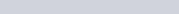Unlike the unimolecular reaction earlier, this reaction involves three total rate constants. k1 describes the forward rate of the enzyme and substrate forming the complex, while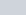describes the reverse reaction.relates to the formation of the product and regeneration of the enzyme from the complex. To simplify the math, we can often implement the steady state approximation as well as another useful approximation related to equilibrium.##### How Steady State fits with Enzymes

Both in biological systems and industrial applications, the substrate tends to exist in vast excess of the enzyme. This fulfills the “low concentration of intermediate” condition since the low enzyme concentration limits the formation of the complex. This alone allows us to assume a steady-state of the enzyme complex since the high concentration of substrate requires essentially all enzymes to be saturated with substrate. This results in the concentration of the enzyme-substrate complex remaining constant for the duration of the reaction: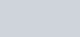##### The Simplified Math

To express the change in concentration of the complex, we use the forward rate of the first reaction minus the reverse rate as well as the forward rate of the second reaction. Importantly, we can derive each of these rates using first and second-order rate laws. We can then use the steady state approximation to relate the three rates:We can then rearrange to yield the following equation: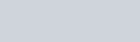At this point, we should remember that [E] and [ES] mathematically relate to one another. Specifically, their addition yields the total concentration of enzyme, used and unused: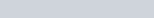Here, we should substitute [E] for [E]total-[ES]: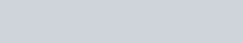The expression on the right equals what chemists call the Michaelis constant. This value has use in characterizing the affinity of enzymes with their substrates. Higher Michaelis constants mean lower affinity, while enzyme-substrate pairs with high affinity have low constants.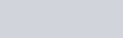Substituting the Michaelis constant into the earlier equation, we can then solve for [ES]:Finally, we can substitute this expression into the rate of product formation. This equation then serves as the initial velocity of an enzymatic reaction:Thus, thanks to the steady state approximation, we can derive a reaction’s initial velocity, an important parameter for biochemists studying enzyme kinetics.

## Steady State Approximation Practice Problems

Problem 1

Find the rate law for the decomposition of N2O5 in the following mechanism. Use the steady state assumption to assume that the rate of change of each intermediate is 0, and perform the necessary substitutions.Problem 2

Find the rate law for the decomposition of O3 in the following mechanism. Use the steady state assumption to assume that the rate of change of O is 0, and perform the necessary substitutions.